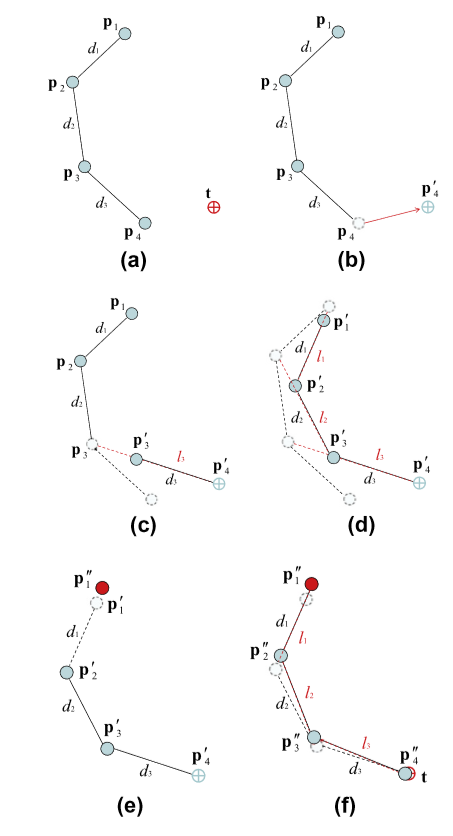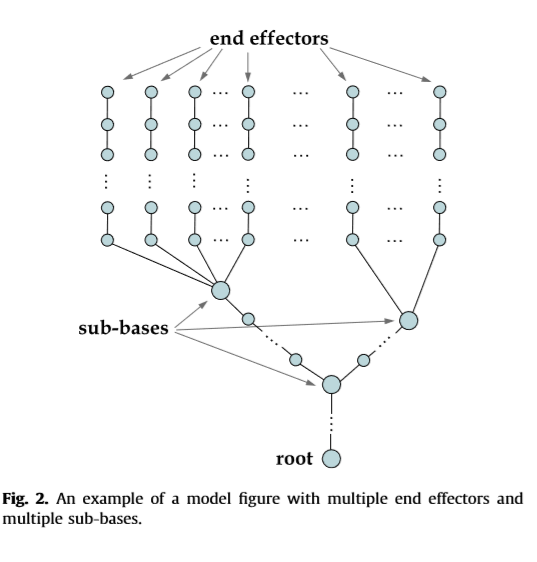# 角色动画研究 —— FABRIK

## FABRIK的工作原理

FABRIK的工作原理其实比较简单，相对于以计算效率见长的CCD来说应该还会更快一些，同时，效果也会更好。对于刚体骨骼的IK计算来说，应该是目前游戏开发这一块比较好用的IK结算算法了。

1. 首先，针对一条从首端到尾端的bone chain，以及一个对应的Target point，先计算整条chain的长度，从而判断这个Target point是否可到达。
1.1 如果不可到达，则要求根骨骼进行移动。通常的情境类似于一个人如果伸直了手或者一只长颈鹿伸长了脖子也无法够到目标点时，则此时需要自己本身就向目标点挪（在使用Jacobian矩阵计算的环境下，此时矩阵为irregular，无对应的逆可以算出来）。
1.2 如果可以到达，那么这就意味着可以进行下一步的处理了，这里假设一共有五根骨骼（从根骨骼到末端骨骼分别是${p}_{0}$$p_0$, ${p}_{1}$$p_1$,${p}_{2}$$p_2$, ${p}_{3}$$p_3$,${p}_{4}$$p_4$），与目标位置(t)。

2. 如果整条骨骼链可以保证够得着的话，那么就可以开始进行FABRIK的处理。整体分为两步，首先，先从末端骨骼开始计算，先将最末端的骨骼${p}_{4}$$p_4$移到目标位置t处，此时骨骼${p}_{4}$$p_4$的位置为${p}_{4}^{{}^{\prime }}$$p_4^{'}$

3. 然后，将${p}_{3}$$p_3$${p}_{4}^{{}^{\prime }}$$p_4^{'}$连成一条直线，通过原有的${p}_{3}$$p_3$${p}_{4}$$p_4$的距离，将现在的${p}_{3}$$p_3$拉到与${p}_{4}^{{}^{\prime }}$$p_4^{'}$同样的距离处${p}_{3}^{{}^{\prime }}$$p_3^{'}$
4. 如是者三，一直处理到根骨骼${p}_{0}$$p_0$
5. 再之后，再从根骨骼${p}_{0}^{{}^{\prime }}$$p_0^{'}$开始处理。由于根骨骼再整个迭代过程中是默认为不动的，因此再把根骨骼${p}_{0}^{{}^{″}}$$p_0^{''}$移到原来的位置${p}_{0}$${p_0}$处，接着使用同样的距离约束，一直处理到尾骨骼${p}_{4}$$p_4$
6. 重复2~5的迭代过程，直到最终的尾骨骼位置与到达目标位置（或与目标位置距离小于某个预定值）停止。此时整个算法结束。## 多端约束与环形约束## 骨骼约束

FABRIK的骨骼约束其实与一般的基于iteration的骨骼约束差不多，并且针对工程来讲，还是统一的骨骼约束方案比较现实，因此这里并不展开讲。如果以后有时间可以另起一篇博客进行讲解。

## 实际运用

FABRIK已经在UE4中被封装成了节点，可以针对链条骨骼模型、树状骨骼模型等直接进行操作，读者可以直接进行使用。如果感兴趣，可以阅读其源码。

<全文完>